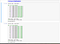Pytorch vs numpy.

Before discussing the topic, for those users who don’t know about pytorch, it is a Python-based scientific computing package. You can read more about it here :- https://pytorch.org/

I will describe the similarities between the pytorch, and numpy and also compare their execution rates. Kinda like benchmark.

1) Performance at creating uninitialized array :-

Both numpy, as well as pytorch are beast in mathematical computing. But, numpy is slightly better than pytorch. This difference however can end up being a decisive edge in real time systems.Empty array initilization in numpy, and pytorch.

As seen in the above code, I have initialized 14 arrays of size 40000 X 40000, one million times. And, numpy is clearly better, than pytorch in large scale computation. For, small-scale computation, both performs roughly the same.

Also, pytorch has a function named ‘zeros’, but it’s very computationally heavy(so heavy, it’s out of scope for 16 gigs of ram) than numpy’s zero function. Hence, clearly numpy is more efficient, and fast in array initialization.

Also note, numpy perform operation at ‘array’ datatype, and pytorch performs operations at ‘tensor’ datatype.

2) Performance at Array Operations :-

In terms of array operations, pytorch is considerably fast over numpy. Both are computationally heavy.

As we see pytorch is faster than numpy in mathematical operations over 10000 X 10000 matrices. This is because of faster array element access that pytorch provides.

3) Array traversing.

Here, I have taken a one dimensional vector having size 10 billion random elements. And, I have tried to access the middle element from both numpy, as well as pytorch.

The result is decisive, pytorch is clearly a winner in array traversing. It took about 0.00009843 seconds in pytorch, while over 0.01 seconds for numpy!

Conclusion :-

Pytorch is a fantastic library, and is widely used in research for testing, and trying new ML, and deep learning models. Numpy on other hand is more stable than pytorch as it has been in development for longer period.

According to these benchmarks, pytorch is clear winner for array operations, and traversing. One of the factors for faster operations in pytorch could be because of faster array element access.

However pytorch could sometime be more computationally heavy for the RAM, and may require more gigs to implement the models which could had been implemented in lesser memory in numpy.

Also, they both use different core datatypes. The ‘tensors’ are widely used in models implemented in pytorch, and using numpy array may become complex.

Ultimately, you would take decision on your requirements, do you want to build a pytorch model? If yes, then do mathematical operations in pytorch. Do you want to build a model manually, or via tensorflow? If yes, then do the mathematical operations on numpy.

Inter conversion of these datatype is both computationally heavy, and complex. And it is best to avoid doing so.

EDIT :-

Edit 1- Rectified some critical errors related to considering false time taken due to random number generation, thanks to Eric Wieser.

Written by

Neel Mishra

A place to read and write about all things Python. If you want to become a writer for this publication then let me know.

Welcome to a place where words matter. On Medium, smart voices and original ideas take center stage - with no ads in sight. Watch
Follow all the topics you care about, and we’ll deliver the best stories for you to your homepage and inbox. Explore
Get unlimited access to the best stories on Medium — and support writers while you’re at it. Just \$5/month. Upgrade